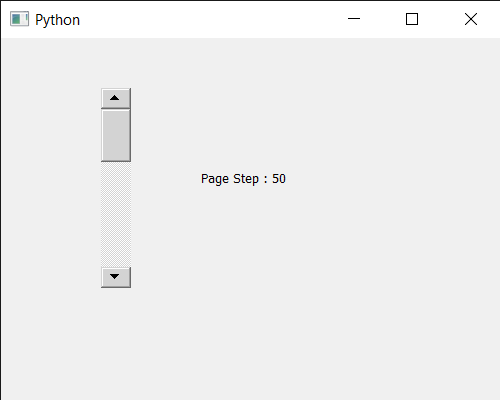# PyQt5 QScrollBar – Getting Page Step

• Last Updated : 30 Jul, 2020

In this article we will see how we can get page step property of QScrollBar. QScrollBar is a control that enables the user to access parts of a document that is larger than the widget used to display it. Slider is the scroll-able object inside the bar.This property holds the single step. This property holds the page step. The larger of two natural steps that an abstract slider provides and typically corresponds to the user pressing PageUp or PageDown. For scroll bar page step increases the size of the slider up to some point, it can be set with the help of `setPageStep` method.

In order to do this we will use `pageStep` method with the scroll bar object.

Syntax : scroll.pageStep()

Argument : It takes no argument

Return : It returns integer

Below is the implementation

 `# importing libraries``from` `PyQt5.QtWidgets ``import` `*` `from` `PyQt5 ``import` `QtCore, QtGui``from` `PyQt5.QtGui ``import` `*` `from` `PyQt5.QtCore ``import` `*` `import` `sys`` ` ` ` `class` `Window(QMainWindow):`` ` `    ``def` `__init__(``self``):``        ``super``().__init__()`` ` `        ``# setting title``        ``self``.setWindowTitle(``"Python "``)`` ` `        ``# setting geometry``        ``self``.setGeometry(``100``, ``100``, ``500``, ``400``)`` ` `        ``# calling method``        ``self``.UiComponents()`` ` `        ``# showing all the widgets``        ``self``.show()`` ` ` ` ` ` `    ``# method for components``    ``def` `UiComponents(``self``):`` ` `        ``scroll ``=` `QScrollBar(``self``)`` ` `        ``# setting geometry of the scroll bar``        ``scroll.setGeometry(``100``, ``50``, ``30``, ``200``)`` ` `        ``# making its background color to green``        ``scroll.setStyleSheet(``"background : lightgrey;"``)`` ` `        ``# setting single step``        ``scroll.setPageStep(``50``)`` ` `        ``# creating a label``        ``label ``=` `QLabel(``"GeesforGeeks"``, ``self``)`` ` `        ``# setting geometry to the label``        ``label.setGeometry(``200``, ``100``, ``300``, ``80``)`` ` `        ``# making label multi line``        ``label.setWordWrap(``True``)`` ` `        ``# getting value changed signal``        ``scroll.valueChanged.connect(``lambda``: do_action())`` ` `        ``# method called when signal is emitted``        ``def` `do_action():``             ` `            ``# setting text to the label``            ``label.setText(``"Current Value : "` `+` `str``(scroll.value()))`` ` `        ``# getting page step property``        ``value ``=` `scroll.pageStep()`` ` `        ``# setting text to the label``        ``label.setText(``"Page Step : "` `+` `str``(value))`` ` ` ` ` ` `# create pyqt5 app``App ``=` `QApplication(sys.argv)`` ` `# create the instance of our Window``window ``=` `Window()`` ` `# start the app``sys.exit(App.``exec``())`

Output :My Personal Notes arrow_drop_up